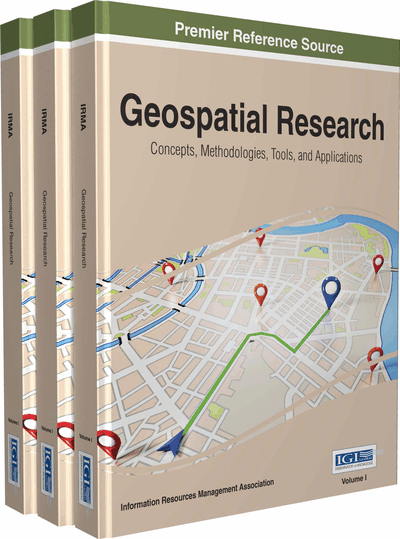# Parallel kNN Queries for Big Data Based on Voronoi Diagram Using MapReduce

Wei Yan (Liaoning University, China)
DOI: 10.4018/978-1-4666-9845-1.ch029
Available
\$37.50
No Current Special Offers

## Abstract

In cloud computing environments parallel kNN queries for big data is an important issue. The k nearest neighbor queries (kNN queries), designed to find k nearest neighbors from a dataset S for every object in another dataset R, is a primitive operator widely adopted by many applications including knowledge discovery, data mining, and spatial databases. This chapter proposes a parallel method of kNN queries for big data using MapReduce programming model. Firstly, this chapter proposes an approximate algorithm that is based on mapping multi-dimensional data sets into two-dimensional data sets, and transforming kNN queries into a sequence of two-dimensional point searches. Then, in two-dimensional space this chapter proposes a partitioning method using Voronoi diagram, which incorporates the Voronoi diagram into R-tree. Furthermore, this chapter proposes an efficient algorithm for processing kNN queries based on R-tree using MapReduce programming model. Finally, this chapter presents the results of extensive experimental evaluations which indicate efficiency of the proposed approach.
Chapter Preview
Top

## Introduction

With the development of the location-based services, the amount of geospatial data is rapidly growing. The nearest neighbor queries are important issue, especially the amount of data is huge with big datasets. In this way, the query requires a lot of time consuming. The Cloud computing enables a considerable reduction in operational expenses. Google’s MapReduce programming model provides a cloud computing platform, which is parallel query processing for big datasets. Given the available cloud services and parallel geospatial queries, a variety of geospatial queries can be modeled using MapReduce programming model. This chapter proposes a method of parallel kNN queries for big dataset based on Voronoi diagram using MapReduce programming model.

The k-nearest neighbor query (kNN) is an important problem that has been frequently used, due to numerous applications including knowledge discovery, pattern recognition, and spatial databases. Given a data set S and a query set R, the kNN query is k nearest neighbors from points in S for each query point rR. Now, lots of researches (Yao et al. 2010) have been devoted to improve the performance of kNN query algorithms. However, all these approaches focus on methods that are to be executed on multi-dimensional data sets. In multi-dimensional data sets the kNN query is complex, and its efficiency is low. How to perform the kNN query on two-dimensional data sets is an important topic in cloud computing environments.

Previous work has concentrated on the spatial databases. In the solution methods the database engine is necessary. For example, new data index and query algorithms need to be incorporated into the database engine. This requirement poses the introduction of R-trees (Guttman 1984), which indexes multi-dimensional data and develops novel algorithms based on R-trees for various forms of Nearest Neighbor (NN) queries. All these approaches focus on methods that are to be executed in a single thread on a single machine. With the quick increase in the scale of the input datasets, processing big data in parallel and distributed database systems is becoming a popular practice.

Parallel spatial query processing has been studied in parallel database, cluster systems as well as cloud computing platform. In cloud computing environments, a large part of data-processing using MapReduce (Dean et al. 2004) programming model runs extensively on Hadoop. The MapReduce programming model provides a powerful parallel and distributed computing paradigm. A few recent studies construct R-tree index with MapReduce programming model (Cary et al. 2009), but these studies can not support any type of query. A data structure that is extremely efficient in exploring a local neighborhood in a geometric space is Voronoi diagram (Okabe et al. 2000). Given a set of points, a general Voronoi diagram uniquely partitions the space into disjoint regions. The region corresponding to a point p covers the points in space that are closer to p than to any other point.

This chapter presents an approximate algorithm using MapReduce programming model that is based on mapping multi-dimensional data sets into two-dimensional data sets, and transforming kNN query into a sequence of two-dimensional point searches. This chapter uses a small number of random vectors to shift the multi-dimensional data using space-filling z-curves. The z-curves can preserve the spatial locality, and map multi-dimensional data into two-dimensional data. Then, in two-dimensional space this chapter proposes a partitioning method using Voronoi diagram, which incorporates the resulting data into the R-tree index structure. Furthermore, this chapter proposes an efficient algorithm for processing kNN queries based on R-tree using MapReduce programming model.

The objectives of the chapter are summarized as follows:

## Complete Chapter List

Search this Book:
Reset